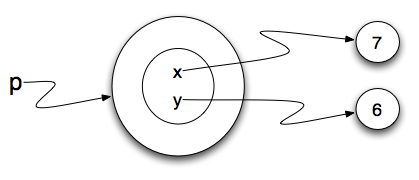# 20.4. Adding Parameters to the Constructor¶

Our constructor so far can only create points at location `(0,0)`. To create a point at position (7, 6) requires that we provide some additional capability for the user to pass information to the constructor. Since constructors are simply specially named functions, we can use parameters (as we’ve seen before) to provide the specific information.

We can make our class constructor more generally usable by putting extra parameters into the `__init__` method, as shown in this example.

```class Point:
""" Point class for representing and manipulating x,y coordinates. """

def __init__(self, initX, initY):

self.x = initX
self.y = initY

p = Point(7,6)
```

Now when we create new points, we supply the x and y coordinates as parameters. When the point is created, the values of `initX` and `initY` are assigned to the state of the object, in the instance variables x and y.

This is a common thing to do in the `__init__` method for a class: take in some parameters and save them as instance variables. Why is this useful? Keep in mind that the parameter variables will go away when the method is finished executing. The instance variables, however, will still be accessible anywhere that you have a handle on the object instance. This is a way of saving those initial values that are provided when the class constructor is invoked.

Later on, you will see classes where the `__init__` method does more than just save parameters as instance variables. For example, it might parse the contents of those variables and do some computation on them, storing the results in instance variables. It might even make an Internet connection, download some content, and store that in instance variables.1. Create a class called `NumberSet` that accepts 2 integers as input, and defines two instance variables: `num1` and `num2`, which hold each of the input integers. Then, create an instance of `NumberSet` where its num1 is 6 and its num2 is 10. Save this instance to a variable `t`.